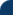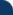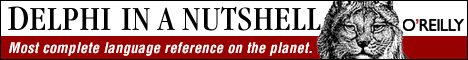Delphi Clinic C++Builder Gate Training & Consultancy Delphi Notes Weblog Dr.Bob's WebshopUnder The Hood #3 - Open Arrays See Also: Delphi Papers and Dr.Bob's Examines Columns

Open parameters is a feature of Delphi that allows string and arrays of varying sizes to be passed to the same procedure or function without violating the strong type checking of the ObjectPascal language. Note that Open Strings are only supported by Delphi 1, since Delphi 2 now supports its own Long Strings (see the previous Under The Hood column).

Delphi 1 Open Strings
Open-string parameters are one step further from the original Borland Pascal attempt. They can be declared in two ways, either by using the OpenString identifier or with the String type in combination with the {\$P+} compiler option (Borland Pascal had a {\$V} compiler switch which is comparable, but not compatible with the new open strings functionality). For an open-string parameter, the actual parameter given to a procedure or function can be of any string size. Within the procedure or function, the maximum length of the formal parameter will be the same as that of the actual parameter. Delphi will take care of possible 'overflow' situation by truncating the strings where necessary (without the danger of a stack overflow, with the {\$V} option in the Borland Pascal days).

```  procedure CopyStr(var OutStr,InStr: OpenString);
begin
OutStr := InStr
end {CopyStr};
```
In the example above, the InStr will be truncated to the maximum allowed length of OutStr, which can be seen in the next example:
```  program Hood3;
uses WinCrt;

procedure CopyStr(var OutStr,InStr: OpenString);
begin
OutStr := InStr
end {CopyStr};

var S1: String;
S2: String;
begin
S1 := 'This is an OpenString Example!';
CopyString(S2,S1);
writeln(S1) { S1 = 'This is an OpenString' }
end.
```
Open-string parameters behave like normal variable parameters of type string, except that they cannot be passed as regular variable parameters to other procedures or functions. They can only be passed as open-string parameters again.

Delphi Open Arrays
A very nice feature of ObjectPascal (in all versions of Delphi) is to use Open Arrays to pass an array to a routine where the routine does not know on forehand exactly how much elements are in this array. The elements of the Open Array are numbered starting with 0 (just like C/C++ and the PChars). Using the High function on an Open Array, we get the number of elements minus one. High always contains the highest possible valid index number, and is -1 is the OpenArray is empty. OpenArrays enable us to write one routine that is capable of accepting arrays of several different lengths, thereby reducing code size! Consider for example the following function Min, which returns the minimum value of an Open Array of Integers:

```  program Hood3;
{\$IFDEF WIN32}
{\$APPTYPE CONSOLE}
{\$ELSE}
uses WinCrt;
{\$ENDIF}

function Min(A: Array of Integer): Integer;
var
i: Word;
begin
Result := High(Integer);
for i := Low(A) to High(A) do { note: Low(A) is always 0! }
if A[i] < result then Result := A[i]
end {Min};

begin
writeln(Min());
writeln(Min([8,4,2,6,5,3,7,12]));
end.
```
The example function Min contains a possible fatal error: the Open Array is supplied as value parameter to Min. This not only costs a lot of time copying the Open Array to the stack, it might also blow the stack! A "value" Open Array is copied onto the stack before the routine is executed (including any stack checking code), and since an Open Array can be up to 64Kb in size (just like the Stack in 16-bits Windows), this offers lots of RunError(202) opportunities. Hence, I urge you to always use Const or Var Open Arrays. They not only save time, they also prevent stack overflows! Like open-string parameters, open-array parameters can only be passed as open-array parameters again.

Delphi 2.0 Bug
Finally, in case you think the Min function from the Open Array example is too obvious to be useful, if you have Delphi Developer 2 (or the C/S version), check the sources directory for the source code of the MATH unit. This unit contains high-performance arithmetic, trigonometric, logarithmic, statistical and financial calculation routines which supplement the math routines that are part of the Delphi language or System unit. Inside the MATH unit, you'll find two routines called MinValue and MaxValue, that are implemented as follows:

```  function MinValue( Data: array of Double): Double;
{ MinValue: Returns the smallest signed value in the data array (MIN) }
var I: Integer;
begin
Result := Data[Low(Data)];
for I := Low(Data) + 1 to High(Data) do
if Result < data[i] then Result := Data[I];
end;

function MaxValue(const Data: array of Double): Double;
{ MaxValue: Returns the largest signed value in the data array (MAX) }
var I: Integer;
begin
Result := Data[Low(Data)];
for I := Low(Data) + 1 to High(Data) do
if Result > Data[I] then Result := Data[I];
end;
```
Have you found the problem? Try it with some sample data and you'll see that the MinValue actually returns the largest signed value and the MaxValue returns the smallest signed value. Quite the opposite as was intended. Of course, at the time of writing, an update of Delphi 2 was imminent, so these bugs may have been fixed in the current inline release of Delphi Developer 2.01 (although I would advise you to check before trying)...